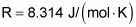##### Physics I For Dummies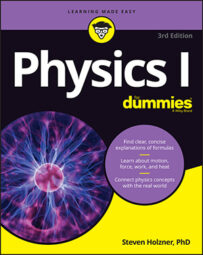Physics involves a lot of calculations and problem solving. Having on hand the most frequently used physics equations and formulas helps you perform these tasks more efficiently and accurately. This Cheat Sheet also includes a list physics constants that you’ll find useful in a broad range of physics problems.

## Physics equations and formulas

Physics is filled with equations and formulas that deal with angular motion, Carnot engines, fluids, forces, moments of inertia, linear motion, simple harmonic motion, thermodynamics, and work and energy.

Here’s a list of some important physics formulas and equations to keep on hand — arranged by topic — so you don’t have to go searching to find them.

## Angular motion

Equations of angular motion are relevant wherever you have rotational motions around an axis. When the object has rotated through an angle of θ with an angular velocity of ω and an angular acceleration of α, then you can use these equations to tie these values together.

You must use radians to measure the angle. Also, if you know that the distance from the axis is r, then you can work out the linear distance traveled, s, velocity, v, centripetal acceleration, ac, and force, Fc. When an object with moment of inertia, I (the angular equivalent of mass), has an angular acceleration, α, then there is a net torque Στ.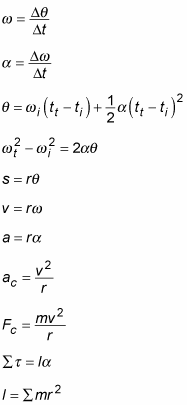## Carnot engines

A heat engine takes heat, Qh, from a high temperature source at temperature Th and moves it to a low temperature sink (temperature Tc) at a rate Qc and, in the process, does mechanical work, W. (This process can be reversed such that work can be performed to move the heat in the opposite direction — a heat pump.) The amount of work performed in proportion to the amount of heat extracted from the heat source is the efficiency of the engine. A Carnot engine is reversible and has the maximum possible efficiency, given by the following equations. The equivalent of efficiency for a heat pump is the coefficient of performance.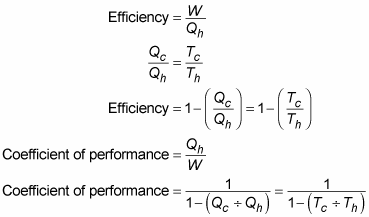## Fluids

A volume, V, of fluid with mass, m, has density, ρ. A force, F, over an area, A, gives rise to a pressure, P. The pressure of a fluid at a depth of h depends on the density and the gravitational constant, g. Objects immersed in a fluid causing a mass of weight, Wwater displaced, give rise to an upward directed buoyancy force, Fbuoyancy. Because of the conservation of mass, the volume flow rate of a fluid moving with velocity, v, through a cross-sectional area, A, is constant. Bernoulli’s equation relates the pressure and speed of a fluid.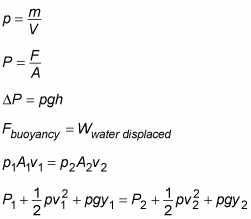## Forces

A mass, m, accelerates at a rate, a, due to a force, F, acting. Frictional forces, FF, are in proportion to the normal force between the materials, FN, with a coefficient of friction, μ. Two masses, m1 and m2, separated by a distance, r, attract each other with a gravitational force, given by the following equations, in proportion to the gravitational constant G: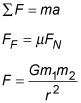## Moments of inertia

The rotational equivalent of mass is inertia, I, which depends on how an object’s mass is distributed through space. The moments of inertia for various shapes are shown here:

• Disk rotating around its center: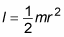• Hollow cylinder rotating around its center: I = mr2

• Hollow sphere rotating an axis through its center: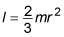• Hoop rotating around its center: I = mr2

• Point mass rotating at radius r: I = mr2

• Rectangle rotating around an axis along one edge where the other edge is of length r: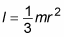• Rectangle rotating around an axis parallel to one edge and passing through the center, where the length of the other edge is r: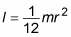• Rod rotating around an axis perpendicular to it and through its center:• Rod rotating around an axis perpendicular to it and through one end:• Solid cylinder, rotating around an axis along its center line:• The kinetic energy of a rotating body, with moment of inertia, I, and angular velocity, ω: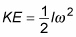• The angular momentum of a rotating body with moment of inertia, I, and angular velocity, ω: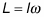## Linear motion

When an object at position x moves with velocity, v, and acceleration, a, resulting in displacement, s, each of these components is related by the following equations: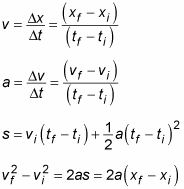## Simple harmonic motion

Particular kinds of force result in periodic motion, where the object repeats its motion with a period, T, having an angular frequency, ω, and amplitude, A. One example of such a force is provided by a spring with spring constant, k. The position, x, velocity, v, and acceleration, a, of an object undergoing simple harmonic motion can be expressed as sines and cosines.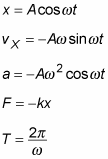## Thermodynamics

The random vibrational and rotational motions of the molecules that make up an object of substance have energy; this energy is called thermal energy. When thermal energy moves from one place to another, it’s called heat, Q. When an object receives an amount of heat, its temperature, T, rises.

Kelvin (K), Celsius (C), and Fahrenheit (F) are temperature scales. You can use these formulas to convert from one temperature scale to another: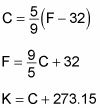The heat required to cause a change in temperature of a mass, m, increases with a constant of proportionality, c, called the specific heat capacity. In a bar of material with a cross-sectional area A, length L, and a temperature difference across the ends of ΔT, there is a heat flow over a time, t, given by these formulas: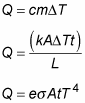The pressure, P, and volume, V, of n moles of an ideal gas at temperature T is given by this formula, where R is the gas constant: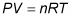In an ideal gas, the average energy of each molecule KEavg, is in proportion to the temperature, with the Boltzman constant k: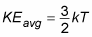## Work and energy

When a force, F, moves an object through a distance, s, which is at an angle of Θ,then work, W, is done. Momentum, p, is the product of mass, m, and velocity, v. The energy that an object has on account of its motion is called KE.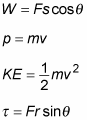## A list of physics constants

Physics constants are physical quantities with fixed numerical values. The following list contains the most common physics constants, including Avogadro’s number, Boltzmann’s constant, the mass of electron, the mass of a proton, the speed of light, the gravitational constant, and the gas constant.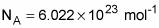• Boltzmann’s constant: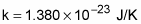• Mass of electron: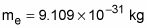• Mass of proton: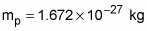• Speed of light: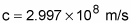• Gravitational constant: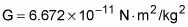• Gas constant: